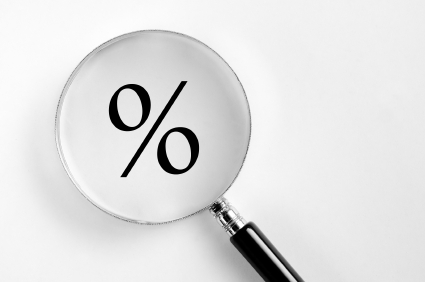# Expert Advice on Hospitality Topics

By Elizabeth Godsmark
Atlantic Publishing

### The Basic Mathematics of ProfitabilityA typical beverage operation generates a constant stream of data and information, endless columns of figures and daily records. But you'd be surprised how few managers actually do anything with these figures, let alone fully grasp their implications. So how can you tell if you're operating profitably? The answer is you can't, unless, of course, you get to grips with some basic mathematics. For a start, you'll need to know how to perform a few simple calculations, such as working out an item's cost percentage. You don't need to be a mathe­matician to figure the following straightforward formulas:

• Cost per ounce. This is the basic unit cost of a drink. For example, to calculate the cost per ounce of a liter bottle, divide the wholesale cost of the bottle by 33.8 ounces, or in the case of a 750ml bottle, by 25.4 ounces. The figure you arrive at is the cost per ounce.
• Cost per portion. To be able to price a certain drink, you must first calculate the base cost of the serving. Use the cost per ounce to work out the cost per portion. For example, if the cost per ounce is \$0.60 and the recipe requires 1.5 ounces, then the portion cost is \$0.90.
• Cost percentage. Master this formula. You cannot function without it! To calculate the cost percentage of an item, divide the product's cost (or portion's cost) by its sale price and then multiply by 100. This simple calculation gives you the cost percentage. Profitability hangs on this key calculation. This calculation is the most frequently used formula in the beverage industry. It indicates the profit margin of any drink and represents the difference between the cost of the item and the price for which it is sold. If cost percentage increases, profit margins decrease..

### Measuring Bottle Yield

You know the theory: to obtain the cost per ounce, you must divide the cost of the bottle by the number of ounces in the bottle. Fine, so far. But sometimes, in practice, the final sales volumes and profits can seem disappointing. You're confused because you have done everything by the book, and now, somehow, the figures don't quite add up. Get wise.

• Consider evaporation and spillage. When calculating a bottle's cost per ounce, the secret is to deduct an ounce or two up front, before dividing, to allow for evaporation or spillage. Although this will slightly increase the cost per ounce, it will also give you a more realistic starting point.
• Calculation errors. Slight variations can easily creep into a calculation involving both liters and ounces. For example, assume a highball contains 1-1/2 ounces of spirit (or 45ml): using ounces, a liter bottle yields 22.54 measures, whereas, using milliliters, the bottle gives 22.22 measures. Tip: "round down" in the interests of reality.
• Maximize potential yield. You know that a bottle of liquor yields so many measures at a certain cost. However, you also know that sloppy pouring methods can wipe out potential profits. The best way to overcome this problem is to standardize portion serving as much as possible. You've paid for the liquor and want maximum returns.
• Buy big. High-turnover liquor, wines and spirits should always be purchased in larger bottles for better yield per measure.

### Gross Profits: The Lowdown

There is no better indicator of a business's success than its gross profit figure. By definition, gross profit is the cash difference between an item or portion cost and its sales price. All attempts to reduce costs should focus on this gross profit figure. Get to grips with how to figure out some important calculations related to gross profits.

• Gross profit. To calculate a drink's gross profit, simply subtract its portion cost from its sale price.
• Gross profit margin. This figure represents the percentage amount of profit made by the sale. Divide the amount of profit by the sales price and then multiply by 100. The result is the gross profit margin.
• Sales percentage profits. To calculate the selling price (based on the required gross profit margin), divide the portion cost by the gross profit margin percentage "reciprocal," i.e., the figure you get from subtracting the target gross margin from 100.
• Cost multiplier. This calculation is often used in the beverage industry to figure out the target selling price for a drink based on its portion cost. Divide the cost percentage you require by 100 and then multiply the result by the portion cost of the product.
• Mixed-drink prime ingredient costing. A calculation used to determine the target sales price for a mixed drink that has only one main ingredient, such as gin and tonic or scotch on the rocks. All you have to do is divide the drink's portion cost by the target cost percentage.